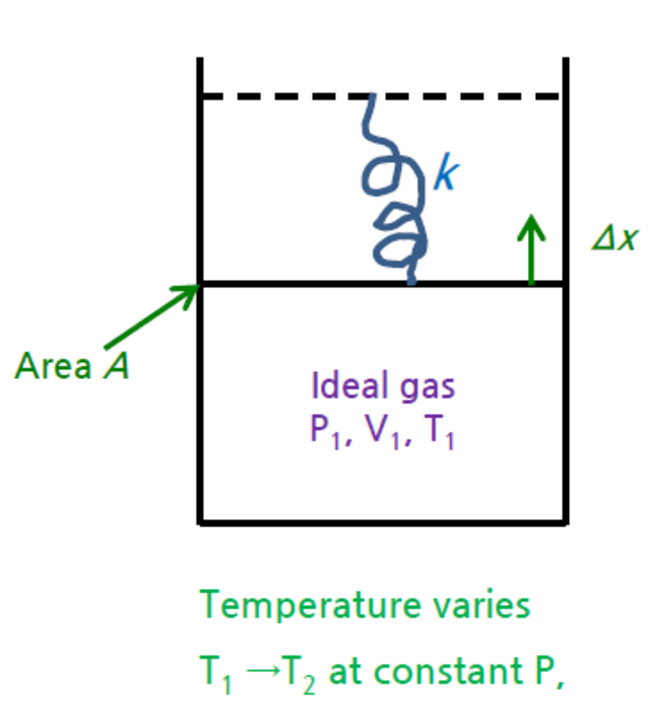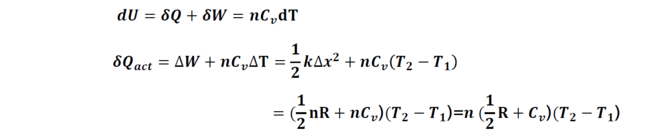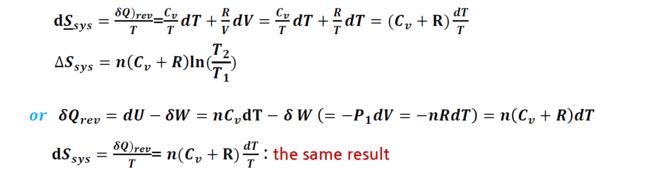## Want to keep learning?

This content is taken from the Hanyang University's online course, Thermodynamics in Energy Engineering. Join the course to learn more.
4.8

# Example : Entropy of gas spring system

### In the spring-gas system beforeAn ideal gas is contained in a cylinder. Initially the pressure, volume and temperature were P1, V1, and T1. A spring with a spring constant k attaches to the piston as the figure. The area of piston is A. The gas expands against the spring by increasing temperature to at constant pressure T2. Calculate the entropy change of the ideal gas subsystem.

As an exercise, let’s calculate the actual heat first. From the article in week 2( 'Example 2: Equation of state for ideal gas'), we know that the displacement has a relationship k∆x2=nR(T2−T1).

• Whole system : closed
• Subsystem 1: ideal gas, Rest: surrounding

#### For ideal gas subsystem 1Entropy change of the ideal gas subsystem :
Take a reversible path = reversible expansion at constant pressure